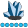Bal23
Lapis Lazuli | Level 10

## How to get F value

I need to use reduction operator to get F value for a dataset, that contains one coninous variable (y) and one categoricacl variable (x), whith three levels. my continous dependent variable and categorical independent variable in my single factor anova model

now I need to use sas iml to get sum of squares (SS), with this, I can calculate F value, MSmodel/MSerror.

can anybody provide a sample code? Thanks.

6 REPLIES 6

## Re: How to get F value

Is there a particular reason to be using IML instead of SAS Procs?

## Re: How to get F value

Here is one possible way using the DESIGN function.

``````  y = y - y[:];
d = design(x);
n = nrow(y);
m = ncol(d);
r = ssq(y - d * ((d / d[+,])` * y) );
F = (( y[##] - r)/(m - 1)) / (r/(n - m));``````Bal23
Lapis Lazuli | Level 10

## Re: How to get F value

ThanksBal23
Lapis Lazuli | Level 10

## Re: How to get F value

is x independent variable, y dependent variable?  My y variable is charcter variable, should I change it to "1,2,3" as it has three groups so that the code can be used? Or is there an alternative way to run it? Thanks.

736 proc iml;

737 reset print;

738 use have;

739 read all var {useful} into z;

WARNING: No output destinations active.

740 read all var {useful} into x1 where(tem="low");

741 read all var {useful} into x2 where(tem="mid");

742 read all var {useful} into x3 where(tem="war");

743 read all var {tem} into y;

744 print y;

745

746 x=x1||x2||x3;

statement : ASSIGN at line 769 column 3

770 F = (( y[##] - r)/(m - 1)) / (r/(n - m));

ERROR: Character operation not implemented yet.

ERROR: (execution) Unknown or error operation executed.

operation : [ at line 770 column 11

operands : y, \$SUB0008

y 33 rows 1 col (character, size 3)

statement : ASSIGN at line 770 column

## Re: How to get F value

In your original message you stated that the data "contains one continous variable (y) and one categoricacl variable (x) with three levels."  Now you are saying that y is character with three levels. Which is it?

In the code that Ian provided, the X variable can be numeric or character because the DESIGN function always returns a numerical matrix. However, the Y variable must be numerical to make sense of the centering operation (y - y[:]), sum of squares (y[##]), and the other arithmtic operations.

We are very happy to provide you with assistance, but please state the conditions of your problem clearly and accurately, including the nature of the data.

## Re: How to get F value

I agree with Rick, the more clearly and carefully you state the problem, the more likely you are to get useful help.

In my code above, both x (categorical) and y (numeric) are column vectors of the same length, and they do not need to be sorted by category.  It looks like you are trying to make a matrix x with 3 columns which is wrong.   So I am guessing that if you go with:

``````use have;
read all var {useful} into y;
read all var {tem} into x;``````

and then run my code, you should get the F-value that you want.

From The DO Loop